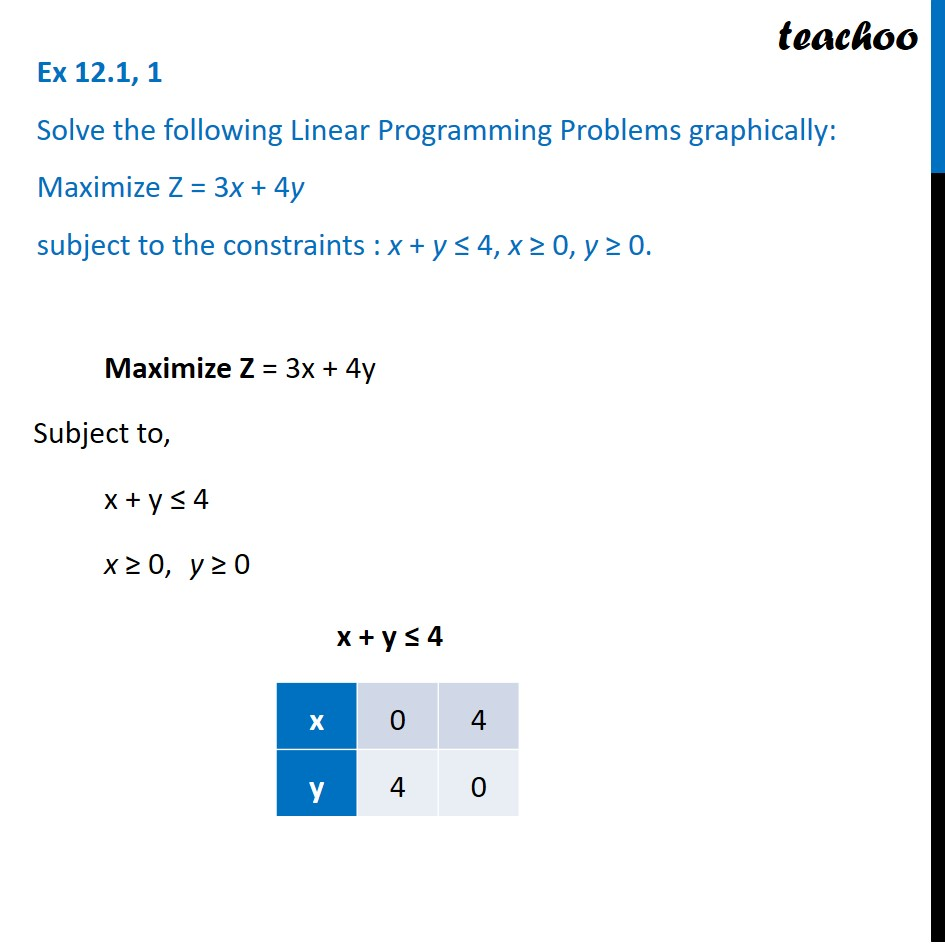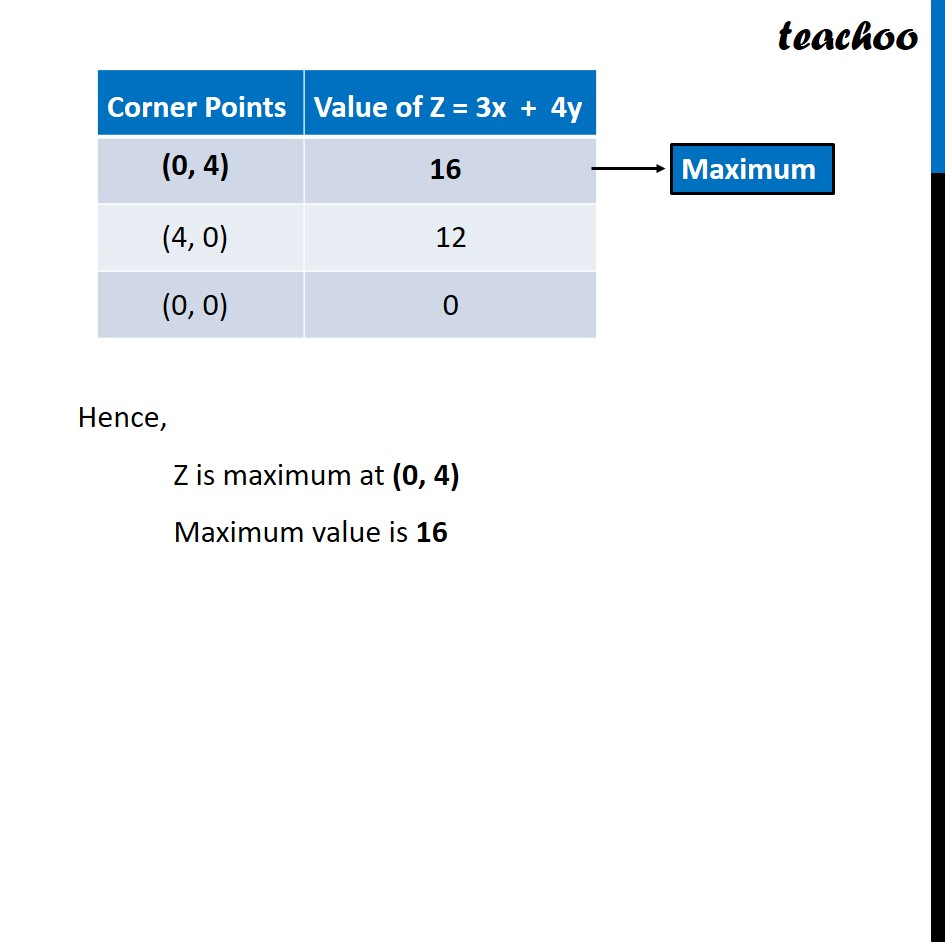Ex 12.1

Chapter 12 Class 12 Linear Programming
Serial order wiseLearn in your speed, with individual attention - Teachoo Maths 1-on-1 Class

### Transcript

Ex 12.1, 1 Solve the following Linear Programming Problems graphically: Maximize Z = 3x + 4y subject to the constraints : x + y ≤ 4, x ≥ 0, y ≥ 0. Maximize Z = 3x + 4y Subject to, x + y ≤ 4 x ≥ 0, y ≥ 0 x + y ≤ 4 Hence, Z is maximum at (0, 4) Maximum value is 16